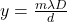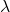## In a double-slit interference experiment, which of the following actions (perhaps more than one) would cause the fringe spacing to increase?

Question

In a double-slit interference experiment, which of the following actions (perhaps more than one) would cause the fringe spacing to increase? (a) Increasing the wavelength of the light. (b) Increasing the slit spacing. (c) Increasing the distance to the viewing screen. (d) Submerging the entire experiment in water

in progress 0
2 weeks 2021-09-05T14:11:39+00:00 1 Answers 0 views 0

The correct option is a , c

Explanation:

Generally the  fringe spacing is mathematically defined asWhere y is the fringe spacing

m is the order of the fringeis the wavelength

D is the distance between the slit and the screen

d is the distance between the slit

Now in order to increase the fringe spacing can do the following

• Increase the wavelength
• increase the distance from the slit to the screen
• Decrease the distance between the slit

The following action would increase the fringe spacing because the from the question these parameters are directly proportional to the fringe spacing